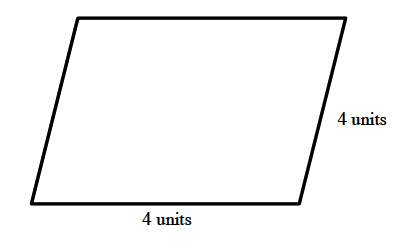### Home > CC1 > Chapter Ch4 > Lesson 4.2.3 > Problem4-70

4-70.

On graph paper, draw any quadrilateral. Then enlarge (or reduce) it by each of the following ratios. Homework Help ✎

The quadrilateral below will be used to show you how to do this problem.
For your homework, use a different quadrilateral and follow the steps and hints shown here.

1. $\quad \frac { 4 } { 1 }$

To enlarge it by 4:1, each side must be four times as long.
In this case, each side of the parallelogram must be four units long.1. $\quad \frac { 7 } { 2 }$

We can start by making this a ratio in the form of

$\frac{x}{1},$

since our original quadrilateral has sides of length 1 unit. Thus we find the new ratio of

$\frac{7}{2}=\frac{3.5}{1}.$

This means each side of the new quadrilateral must be 3.5 times longer than that of the original.